#### 期刊菜单

Research on Optimal Control of Triple Phase Shift Angle Based on MOPSO
DOI: 10.12677/JEE.2021.91002, PDF, HTML, XML, 下载: 216  浏览: 471  科研立项经费支持

Abstract: Dual-active-bridge (DAB) DC/DC converter is widely used for its high power density and flexible operation mode. But it also has the disadvantages of complicated control amount and high return power. However, the complexity of control quantity and high reflux power are the main disadvantages of the system, which has become the bottleneck of its development. In view of the above defects, by analyzing the triple-phase-shift (TPS) control strategy, this paper further analyzes the different effects of the three phase-shifting degrees of freedom on the transmission power and the effective values of the inductance current. In this paper, based on the TPS control strategy, the influence of three degrees of freedom on transmission power and effective value of inductor current is analyzed, and the relationship equation between the transmitted power and the effective values of the inductance current in the TPS control system is designed. Based on the equation, intelligent particle swarm optimization (PSO) is used to optimize the phase shift angle. the method that using intelligent PSO to optimize the three phase shift angle has been proposed, which can improve the convergence speed of the optimal phase shift angle. Simulation and experiments show that this method can improve the transmission power and reduce the effective value of inductance current.

1. 引言

2. 移相比组合优化分析

2.1. 传输功率特性分析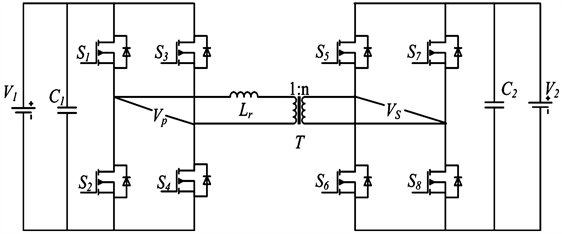Figure 1. DAB converter topology diagram

TPS与传统控制方式相比，控制灵活度增加，传输功率的范围从曲线扩展成曲面。如图1所示DAB，一次侧全桥的输出电压VP受控于D1，二次侧输出电压VS受控于D0、D2。一次侧全桥输出电压VP跟随D1的变化，输出电压VP波形呈现出“0正0负”的变化规律。二次侧全桥输出电压Vs则随着D0、D2的变化而变化，具体来说可能有四种变化规律，即“0正0负”、“0负0正”、“正0负0”、“负0正0”。即在三重移相控制下由于增加桥间移相角，变压器原副边电压形成三电平方波。为简化数学模型，根据以上的变化规律，如图2所示，可将产生的三电平方波可以分解成两个对称的两电平方波。则一次侧全桥输出电压、二次侧全桥输出电压可表示为式(1)。

$\left\{\begin{array}{l}{v}_{p}\left(t\right)={V}_{1}\left(S\left(t\right)+S\left(t-{D}_{1}T\right)\right)\\ {v}_{s}\left(t\right)={V}_{2}\left(S\left(t-{D}_{0}T\right)+S\left(t-{D}_{0}T-{D}_{2}T\right)\right)\end{array}$ (1)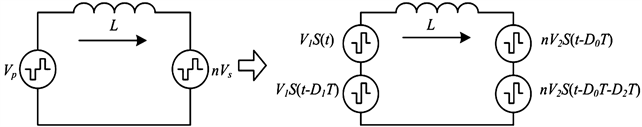Figure 2. DAB equivalent transformation model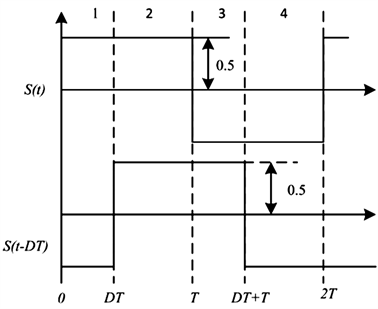Figure 3. Standard two-level waveform

$L\frac{\text{d}{i}_{L}}{\text{d}t}={v}_{p}-n{v}_{s}={V}_{1}\left(S\left(t\right)+S\left(t-{D}_{1}T\right)\right)-n{V}_{2}S\left(t-{D}_{0}T\right)+S\left(t-{D}_{0}T-{D}_{2}T\right)$ (2)

${i}_{L}\left(t\right)=\frac{\int \left[{V}_{1}\left(S\left(t\right)+S\left(t-{D}_{1}T\right)\right)\right]\text{d}t}{L}-\frac{\int \left[n{V}_{2}\left(S\left(t-{D}_{0}T\right)+S\left(t-{D}_{1}T\right)\right)\right]\text{d}t}{L}$ (3)

$P={v}_{p}\left(t\right)\int {i}_{L}\left(t\right)\text{d}t$ (4)

$P=-\frac{n{V}_{1}{V}_{2}}{TL}{\int }_{{D}_{1}T}^{T}\left({\int }_{{D}_{1}T}^{T}\left(S\left(t-{D}_{0}T\right)+S\left(t-{D}_{0}T-{D}_{2}T\right)\right)\text{d}t\right)\text{d}t$ (5)

${I}_{rms}^{2}=\frac{1}{T}{\int }_{0}^{T}{i}^{2}\left(t\right)\text{d}t=\frac{1}{T{L}^{2}}{\int }_{0}^{T}\left({\int }_{0}^{T}\left({V}_{1}\left(S\left(t\right)+S\left(t-{D}_{1}T\right)\right)-n{V}_{2}\left(S\left(t-{D}_{0}T\right)+S\left(t-{D}_{1}T\right)\right)\right)\text{d}t\right)\text{d}t$ (6)Figure 4. Main voltage and current waveforms in six working modesTable 1. Six power transmission mode ranges

$\left\{\begin{array}{l}{P}_{A}=\frac{-{V}_{1}{V}_{2}T}{L}\left(-{D}_{0}+{D}_{0}^{2}+0.5{D}_{1}-{D}_{0}{D}_{1}+0.5{D}_{2}+{D}_{0}{D}_{2}+0.5{D}_{2}^{2}\right)\\ {P}_{E}=\frac{-{V}_{1}{V}_{2}T}{L}\left(-{D}_{0}+0.5{D}_{1}+{D}_{0}{D}_{1}-0.5{D}_{1}^{2}-0.5{D}_{2}+0.5{D}_{1}{D}_{2}\right)\\ {P}_{F}=\frac{-{V}_{1}{V}_{2}T}{L}\left(-{D}_{0}+0.5{D}_{0}^{2}+0.5{D}_{1}-0.5{D}_{2}+{D}_{0}{D}_{2}-0.5{D}_{1}{D}_{2}+0.5{D}_{2}^{2}\right)\end{array}$ (7)

$\stackrel{¯}{P}=\frac{P}{\frac{n{V}_{1}{V}_{2}}{8{f}_{s}L}}$ (8)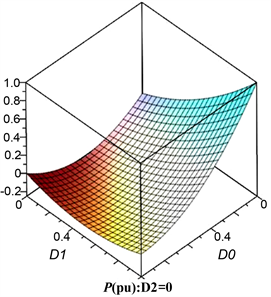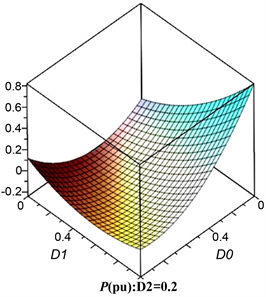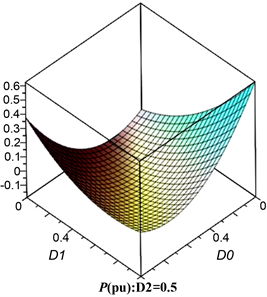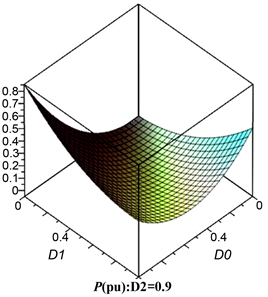Figure 5. Three-dimensional change graph of sub-mode standardized transmission power

2.2. 回流功率特性分析

${t}_{zero}\le \frac{1}{2}\left(1-{D}_{1}\right)T$ 时，回流功率小于等于0。回流功率可表示为：

${P}_{cir}=|-{v}_{p}\left(t\right)\int {i}_{L}\left(t\right)\text{d}t|$ (9)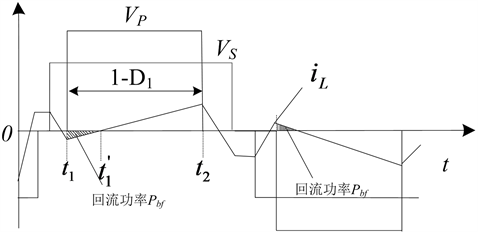Figure 6. Schematic diagram of sub-modal return power

$q=-\left(\frac{3}{8}{D}_{1}^{2}-\frac{1}{4}{D}_{1}-\frac{1}{8}\right){T}^{2}{V}_{1}^{2}+\left(\frac{1}{2}{D}_{0}{D}_{1}-\frac{1}{2}{D}_{0}+\frac{3}{4}{D}_{1}{D}_{2}-\frac{1}{2}{D}_{1}+\frac{1}{4}{D}_{2}-\frac{3}{8}{D}_{1}^{2}-\frac{1}{8}\right){T}^{2}n{V}_{2}{V}_{1}$ (10)

$\Delta q=q\left(D+\Delta d\right)-q\left(D\right)\approx \frac{\text{d}q}{\text{d}D}\Delta d=\frac{\partial q}{\partial {D}_{0}}\Delta {d}_{0}+\frac{\partial q}{\partial {D}_{1}}\Delta {d}_{1}+\frac{\partial q}{\partial {D}_{2}}\Delta {d}_{2}$ (11)

$\left\{\begin{array}{l}\frac{\partial q}{\partial {D}_{0}}=\frac{1}{2}\left({D}_{1}-1\right)<0\\ \frac{\partial q}{\partial {D}_{1}}=-\left(\frac{3}{4}{D}_{1}-\frac{1}{4}\right){T}^{2}{V}_{1}^{2}+\left(\frac{1}{2}{D}_{0}-\frac{1}{2}\right){T}^{2}n{V}_{1}{V}_{2}<0\\ \frac{\partial q}{\partial {D}_{2}}=\left(\frac{3}{4}{D}_{1}+\frac{1}{4}\right){T}^{2}n{V}_{1}{V}_{2}>0\end{array}$ (12)

2.3. 电感电流有效值特性分析

${I}_{rms}=\sqrt{\frac{1}{T}{\int }_{0}^{T}{\left({i}_{L}\left(t\right)\right)}^{2}\text{d}t}$ (13)

${I}_{rms}^{2}=\frac{1}{T}{\int }_{0}^{T}{i}^{2}\left(t\right)\text{d}t=\frac{1}{T{L}^{2}}{\int }_{0}^{T}\left({\int }_{0}^{T}\left({V}_{1}\left(S\left(t\right)+S\left(t-{D}_{1}T\right)\right)-n{V}_{2}\left(S\left(t-{D}_{0}T\right)+S\left(t-{D}_{1}T\right)\right)\right)\text{d}t\right)\text{d}t$ (14)

$\left\{\begin{array}{l}{i}_{L}\left({t}_{0}\right)=-\frac{n{V}_{2}}{4fL}\left(k+2D-1\right)\\ {i}_{L}\left({t}_{1}\right)=\frac{n{V}_{2}}{4fL}\left(k\left(2D-1\right)+1\right)\end{array}$ (15)

$\begin{array}{c}{I}_{RM{S}_{\text{-}}A}^{2}=\frac{{T}^{2}}{{L}^{2}}\left(\frac{0.125}{3}{V}_{1}^{2}+\frac{0.125}{3}{V}_{2}^{2}+\frac{0.5}{3}\left(0.25-1.5{D}_{1}^{2}+{D}_{1}^{3}\right){V}_{1}^{2}-\frac{0.5}{3}\left(0.25-1.5{D}_{0}^{2}+{D}_{0}^{3}\right){V}_{1}{V}_{2}\\ \text{\hspace{0.17em}}-\frac{0.5}{3}\left(0.25-1.5{\left({D}_{0}-{D}_{1}\right)}^{2}+{\left({D}_{0}-{D}_{1}\right)}^{3}{V}_{1}{V}_{2}\right)-\frac{0.5}{3}\left(0.25-1.5{\left({D}_{0}+{D}_{2}\right)}^{2}+{\left({D}_{0}+{D}_{2}\right)}^{3}{V}_{1}{V}_{2}\right)\\ \text{\hspace{0.17em}}-\frac{0.5}{3}\left(0.25-1.5{\left({D}_{0}+{D}_{2}-{D}_{1}\right)}^{2}+{\left({D}_{0}+{D}_{2}-{D}_{1}\right)}^{3}{V}_{1}{V}_{2}\right)+\frac{0.5}{3}\left(0.25-1.5{D}_{2}^{2}+{D}_{2}^{3}\right){V}_{2}^{2}\right)\end{array}$ (16)

$\begin{array}{c}{I}_{RM{S}_{\text{-}}E}^{2}=\frac{{T}^{2}}{{L}^{2}}\left(\frac{0.125}{3}{V}_{1}^{2}+\frac{0.125}{3}{V}_{2}^{2}+\frac{0.5}{3}\left(0.25-1.5{D}_{1}^{2}+{D}_{1}^{3}\right){V}_{1}^{2}-\frac{0.5}{3}\left(0.25-1.5{D}_{0}^{2}+{D}_{0}^{3}\right){V}_{1}{V}_{2}\\ \text{\hspace{0.17em}}-\frac{0.5}{3}\left(0.25-1.5{\left({D}_{1}-{D}_{0}\right)}^{2}+{\left({D}_{1}-{D}_{0}\right)}^{3}{V}_{1}{V}_{2}\right)-\frac{0.5}{3}\left(0.25-1.5{\left({D}_{0}+{D}_{2}\right)}^{2}+{\left({D}_{0}+{D}_{2}\right)}^{3}{V}_{1}{V}_{2}\right)\\ \text{\hspace{0.17em}}-\frac{0.5}{3}\left(0.25-1.5{\left({D}_{1}-{D}_{2}-{D}_{0}\right)}^{2}+{\left({D}_{1}-{D}_{0}-{D}_{2}\right)}^{3}{V}_{1}{V}_{2}\right)+\frac{0.5}{3}\left(0.25-1.5{D}_{2}^{2}+{D}_{2}^{3}\right){V}_{2}^{2}\right)\end{array}$ (17)

$\begin{array}{c}{I}_{RM{S}_{\text{-}}F}^{2}=\frac{{T}^{2}}{{L}^{2}}\left(\frac{0.125}{3}{V}_{1}^{2}+\frac{0.125}{3}{V}_{2}^{2}+\frac{0.5}{3}\left(0.25-1.5{D}_{1}^{2}+{D}_{1}^{3}\right){V}_{1}^{2}-\frac{0.5}{3}\left(0.25-1.5{D}_{0}^{2}+{D}_{0}^{3}\right){V}_{1}{V}_{2}\\ \text{\hspace{0.17em}}-\frac{0.5}{3}\left(0.25-1.5{\left({D}_{1}-{D}_{0}\right)}^{2}+{\left({D}_{1}-{D}_{0}\right)}^{3}{V}_{1}{V}_{2}\right)-\frac{0.5}{3}\left(0.25-1.5{\left({D}_{0}+{D}_{2}\right)}^{2}+{\left({D}_{0}+{D}_{2}\right)}^{3}{V}_{1}{V}_{2}\right)\\ \text{\hspace{0.17em}}-\frac{0.5}{3}\left(0.25-1.5{\left({D}_{0}+{D}_{2}-{D}_{1}\right)}^{2}+{\left({D}_{0}+{D}_{2}-{D}_{1}\right)}^{3}{V}_{1}{V}_{2}\right)+\frac{0.5}{3}\left(0.25-1.5{D}_{2}^{2}+{D}_{2}^{3}\right){V}_{2}^{2}\right)\end{array}$ (18)

${\stackrel{¯}{I}}_{rms}^{2}=\frac{{I}_{rms}^{2}}{\frac{{V}_{1}^{2}}{24{f}_{s}^{2}{L}^{2}}}$ (19)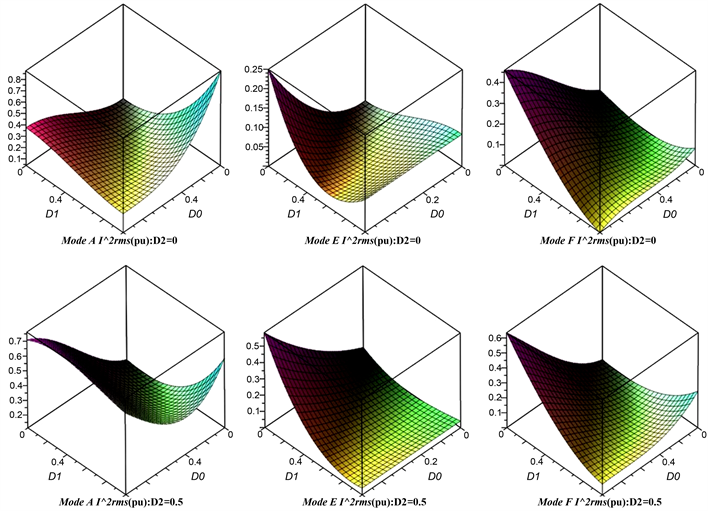Figure 7. The change of the effective value of the inductor current in the three modes

3. 粒子群优化算法

${v}_{i,j}\left(m+1\right)=\omega {v}_{i,j}\left(m\right)+{c}_{1}{r}_{1}\left(pbes{t}_{\iota ,j}\left(m\right)-{x}_{\iota ,j}\left(m\right)\right)+{c}_{2}{r}_{2}\left(gbes{t}_{\iota ,j}\left(m\right)-{x}_{\iota ,j}\left(m\right)\right)$ (20)

${x}_{i,j}\left(m+1\right)={x}_{i,j}\left(m\right)+{v}_{i,j}\left(m+1\right)$ (21)

$\omega =\left({\omega }_{\mathrm{max}}-{\omega }_{\mathrm{min}}\right)\frac{M-m}{M}+{\omega }_{\mathrm{min}}$ (22)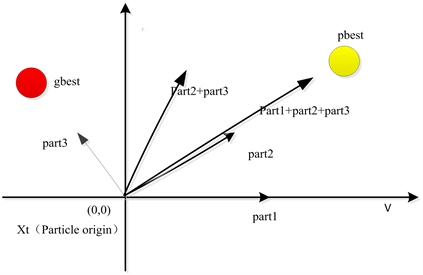Figure 8. Schematic diagram of space particle position change update

$fitness=\lambda \cdot {\stackrel{¯}{P}}_{cir}+\left(1-\lambda \right)\cdot {\stackrel{¯}{I}}_{rms}^{2}$ (23)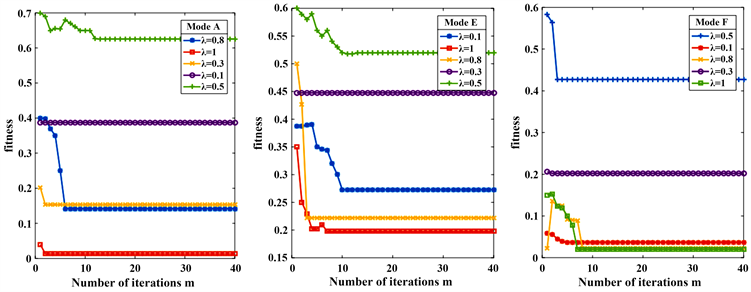Figure 9. Combination optimization of f-phase shift based on different weight coefficients

$\left\{\begin{array}{l}\left\{\begin{array}{l}\mathrm{min}\left[{\stackrel{¯}{P}}_{cir\left(A\right)},{\stackrel{¯}{I}}_{rms\left(A\right)}^{2}\right]\\ 0<{D}_{1}<{D}_{0}<1&{D}_{1}<{D}_{0}+{D}_{2}<1\end{array}\\ \left\{\begin{array}{l}\mathrm{min}\left[{\stackrel{¯}{P}}_{cir\left(E\right)},{\stackrel{¯}{I}}_{rms\left(E\right)}^{2}\right]\\ 0<{D}_{0}<{D}_{1}<1&0<{D}_{0}+{D}_{2}<{D}_{1}\end{array}\\ \left\{\begin{array}{l}\mathrm{min}\left[{\stackrel{¯}{P}}_{cir\left(F\right)},{\stackrel{¯}{I}}_{rms\left(F\right)}^{2}\right]\\ 0<{D}_{0}<{D}_{1}<1&{D}_{1}<{D}_{0}+{D}_{2}<1\end{array}\end{array}$ (24)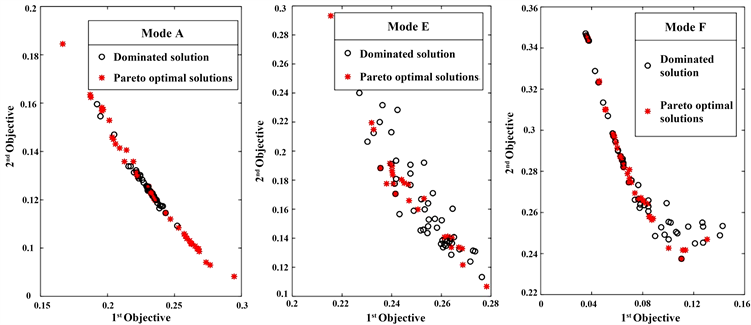Figure 10. Multi-objective optimization based on Pareto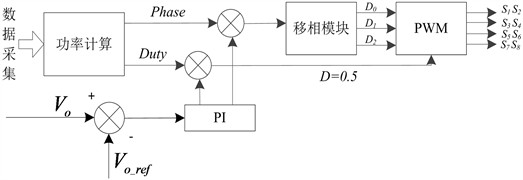Figure 11. Block diagram of DAB converter optimization control realization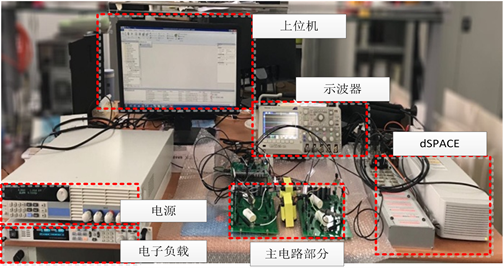Figure 12. Based on hardware-in-the-loop simulation experiment platform system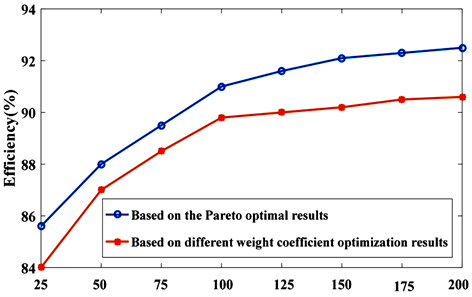Figure 13. Comparison results of two optimization methods

4. 实验结论

  周路遥. 双有源全桥双向DC-DC变换器典型拓扑研究[D]: [硕士学位论文]. 北京: 北京交通大学, 2016.  冯子秋, 迟颂. 双向全桥DC/DC变换器移相优化的扩展双重移相控制[J]. 电气传动, 2019, 49(7): 25-31.  未红旗, 周剑君, 祁良甫. 基于TPS的双向全桥DC/DC最小回流功率控制[J]. 电力电子技术, 2017, 51(10): 14-16.  沙广林. 电力电子变压器中双有源桥DC-DC变换器的研究[D]: [博士学位论文]. 北京: 中国矿业大学(北京), 2016.  武琳, 刘志刚, 洪祥. 隔离式双向全桥DC-DC变换器的功率控制特性比较与分析[J]. 电工技术学报, 2013, 28(10): 179-187.  柳杨. 隔离型双向全桥DC/DC变换器优化控制研究[D]: [硕士学位论文]. 重庆: 重庆大学, 2015.  李良光, 李文君, 朱孟江. UPS双重移相控制的双有源桥DC/DC变换器研究[J]. 电力电子技术, 2019, 53(6): 111-114.  孙孝峰, 吴晓颖, 申彦峰, 崔明勇, 李昕. 一种全功率范围零电压开通的电流型双向隔离DC-DC变换器[J]. 电工技术学报, 2018, 33(10): 2282-2292.  吴俊娟, 孟德越, 申彦峰, 沈虹, 孙孝峰. 双重移相控制与传统移相控制相结合的双有源桥式DC-DC变换器优化控制策略[J]. 电工技术学报, 2016, 31(19): 97-105.  程红, 高巧梅, 朱锦标, 杨小康, 王聪. 基于双重移相控制的双向全桥DC-DC变换器动态建模与最小回流功率控制[J]. 电工技术学报, 2014, 29(3): 245-253.  安峰, 杨柯欣, 王嵩, 罗书聪, 冯晓云. 基于模型前馈的双有源全桥DC-DC变换器电流应力优化方法[J]. 电工技术学报, 2019, 34(14): 2946-2956.  曾进辉, 孙志峰, 雷敏, 兰征. 双重移相控制的双主动全桥变换器全局电流应力分析及优化控制策略[J]. 电工技术学报, 2019, 34(12): 2507-2518.  黄珺, 王跃, 李卓强, 雷万钧. 基于三重移相控制的双主动全桥直流变换器优化调制策略[J]. 中国电机工程学报, 2016, 36(6): 1658-1666.  沙广林, 王聪, 程红, 邓嘉卿, 王健宇. 移相控制的双有源桥DC-DC变换器统一相量分析法[J]. 电工技术学报, 2017, 32(18): 175-185.  程红, 马志鹏, 王聪, 沙广林. 基于傅里叶级数建模的双有源桥DC-DC变换器电流有效值分析[J]. 电力自动化设备, 2017, 37(5): 14-20.  童安平, 杭丽君, 李国杰. 三重移相控制下DAB变换器全局优化控制策略及分析[J]. 中国电机工程学报, 2017, 37(20): 6037-6049.  谷庆, 袁立强, 聂金铜, 李婧, 赵争鸣. 基于开关组合规律的双有源桥DC-DC变换器传输功率特性[J]. 电工技术学报, 2017, 32(13): 69-79.  王凯丽, 张巧杰. MOPSO算法在Boost变换器优化设计中的应用[J]. 烟台大学学报(自然科学与工程版), 2017, 30(4): 317-322.